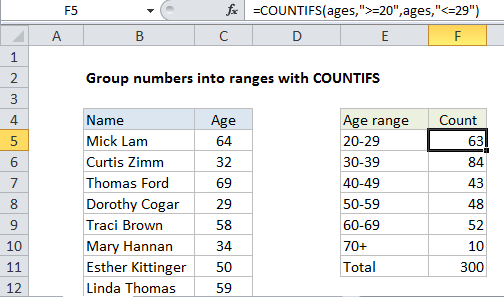## Excel Office

Excel How Tos, Tutorials, Tips & Tricks, Shortcuts

# Count numbers by range with COUNTIFS in Excel

This tutorial shows how to Count numbers by range with COUNTIFS in Excel using the example below;

## Formula

`=COUNTIFS(range,">=low",range,"<=high")`## Explanation

To count numeric data by range or grouping, you can build a summary table and use COUNTIFS to count values at each threshold.

In the example show, we have a list of names and ages and are using the COUNTIFs function to generate a count of ages into 6 brackets. The formula in cell F5 is:

`=COUNTIFS(ages,">=20",ages,"<=29")`

### How this formula works

The named range “ages” refers to C5:C304.

Worked Example:   Summary count of non-blank categories in Excel

The COUNTIFS function lets you count values that meet multiple criteria with an AND relationship (i.e. all criterial must be true).

Worked Example:   Count dates in current month in Excel

In this case, we want to group the data by age range into 10-year brackets, so we use COUNTIFS as follows in column F:

```=COUNTIFS(ages,">=20",ages,"<=29") // 20-29
=COUNTIFS(ages,">=30",ages,"<=39") // 30-39
=COUNTIFS(ages,">=40",ages,"<=49") // 40-49```

For the final bracket, 70+, we only use one criteria:

`=COUNTIFS(ages,">=70") // 70+`

### Dynamic ranges

To expose ranges values on the worksheet where they can be easily changes, you can join references to logical operators with concatenation like this:

`=COUNTIFS(ages,">="\$A1,ages,"<="&B1)`

This formula counts greater than or equal to (>=) the value in A1 and less than or equal to (>=)the value in B1.

Worked Example:   COUNTIFS with multiple criteria and OR logic in Excel

### With a Pivot Table

Pivot Tables offer automatic grouping into equal size ranges.